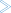# Summary Record Layout

This document describes the format of the summary records copied to a sequential output file. It covers the following topics:

Note:
Software AG does not provide a program that reads this file. However, DSECT samples are supplied in members SUMRECD, SUMRECH and SUMRECS of the source library for users who wish to write their own programs to read this data.

A fixed-length header is created for each record written to the sequential file. The format of the header is described in the following table:

Offset Length Bytes Format Explanation
Hex Decimal
0 0 2 Binary Record Length
2 2 2 Binary X'0000'
4 4 3 Alphanumeric Eye catcher "SUM"
7 7 1 Alphanumeric Record type "H" for header
8 8 32 Alphanumeric Report name
28 40 8 Binary STCK value when record gets written
30 48 1 Binary Flag of trigger event:

X'01' -- report is closed or suspended
X'02' -- time interval reached
X'04' -- trigger command executed
X'08' -- report is closed and restarted

31 49 1 Binary Unused
32 50 10 Alphanumeric Date of first record (YYYY-MM-DD)
3C 60 8 Alphanumeric Time of first record (HH:MM:SS)
44 68 10 Alphanumeric Date of last record (YYYY-MM-DD)
4E 78 8 Alphanumeric Time of last record (HH:MM:SS)
56 86 2 Binary Database ID
58 88 2 Binary Offset to data record
5A 90 6 Binary Unused

## The Schema Portion

This portion of the summary record varies, depending upon the fields used in the report. The schema describes the layout of the field data which follows afterwards. The format of the schema portion of the summary record is shown in the following table:

Offset Length Bytes Format Explanation
Hex Decimal
60 96 2 Binary Record Length
62 98 2 Binary X'0000'
64 100 3 Alphanumeric Eye catcher "SUM"
67 103 1 Alphanumeric Record type "S" for schema
68 106 6 Binary Unused
6E 104 2 Binary Total number of fields
Varies +00 Varies +0 8 Alphanumeric Field name (see the Field Reference)1
+08 +8 2 Binary Data length
+0A +10 2 Binary Number of decimal places. For example, the ADADURA field is displayed in seconds, with six digits after the decimal point. A value of "1.234567" is stored in the data portion of the summary log's summary record as the hexadecimal value `x'0012D687`. In the summary log schema, the number of decimal places is 6.
+0C +12 1 Alphanumeric Data format:

C'B' -- binary
X'C' -- character

Note:
The data format for the DATE field is X'C' (character) for its MIN and MAX values and it is eight bytes long. It contains the date in YYYYMMDD format without any delimiters.

+0D +13 1 Alphanumeric Field type:

C'A' -- Account field
C'C' -- COST field
C'M' -- MIN (minimum) field
C'P' -- PCT (percent) field
C'R' -- RATE field
C'S' -- SUM (summary) field
C'V' -- AVG (average) field
C'X' -- MAX (maximum) field

The COST, MIN, PCT, RATE, SUM, AVG, and MAX fields always have a data length of eight (8) bytes.

1 The following fields use alternate names than the one listed in the field reference list.

Field Name in the Field Reference Field Name in the Summary Record
FILE FNR
IOS IO
NATAPPL LOG
NATPROG PRO
NUCID SMP

#### Determining the Format of the Variable PortionTo determine the format of the variable portion of the record:

• Refer to the report definition for each field (including virtual fields such as summary fields). Twelve bytes in total are reserved for the field name, the data length, the format of the field, and the field type.

## The Data Portion

This portion of the summary record varies, depending upon the fields used in the report. The data portion contains the contents of the fields that are described in the schema portion. The format of the data portion of the summary record is shown in the following table:

Offset Length Bytes Format Explanation
Hex Decimal
Varies +0 Varies +0 2 Binary Record Length
+2 +2 2 Binary X'0000'
+4 +4 3 Alphanumeric Eye catcher "SUM"
+7 +7 1 Alphanumeric Record type "D" for data
+8 +8 Varies Binary/alphanumeric Data portion for all fields, as defined in the schema portion.

#### Determining the Format of the Variable PortionTo determine the format of the variable portion of the record:

• Refer to the schema portion of this record. For each report field, the data length and format are stored.

## Calculating the Number of Summary Records That Can Be Stored

To determine the number of summary records that can be stored on the summary log file, the size of the summary record and the specified block size must be taken into consideration.

The record size of a summary log record can be calculated using the summary record layout described elsewhere in this section. In the following examples, the size of the summary log record is 182 bytes. So the bytes user for one summary record is 186 bytes: `182 + 4` (record length).

#### Example 1: 3390 Device with Block Size of 10.000

• Available bytes per block: `9.996 = 10.000 - 4 =` (4-byte block length)

• Records per block: ```53 = 9.996/186 = Trunc(53,74)```

• Blocks per track: ```5 = 57000/10.000 = Trunc(5,7)```

• Tracks per cylinder: `15`

• Records per cylinder: `3975 = 53 * 5 * 15`

#### Example 2: 3390 Device with Block Size of 27.998

• Available bytes per block: `27.994 = 27.998 - 4 =` (4-byte block length)

• Records per block: ```150 = 27.994/186 = Trunc(150,5)```

• Blocks per track: ```2 = 57000/27.994 = Trunc(2,03)```

• Tracks per cylinder: `15`

• Records per cylinder: `4500 = 150 * 2 * 15`

Comparing these two examples, we see that you can store 525 more records per cylinder when using a larger block size ```(4500 - 3975 = 525)```.